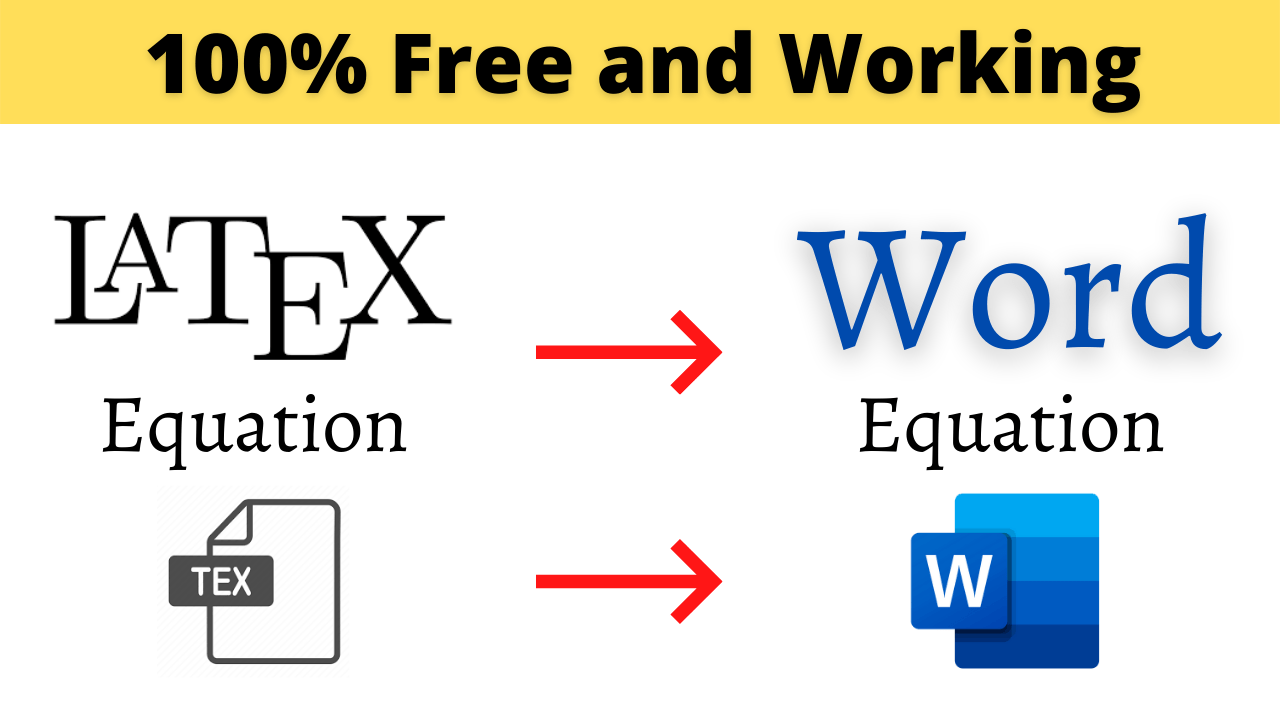# LaTeX equation to Word (2007 to 365): 3 MethodsLaTeX provides lots of features for typing complex mathematical equations with ease compared to other softwares like Ms Word. Microsoft has recognized this and started supporting LaTeX in Office-365. However, if you have an older version of Ms Word you can still bring LaTeX equation into it. This blog covers 3 different ways to bring LaTeX equation in Ms Word.

## 1: Direct LaTeX support in Office 365

Office 365 version 1707 or later allows you to convert LaTeX equation into Ms Words native format (see the following steps). For the older version of Ms Word/Office you can still use Method 2 or Method 3.

### Steps to convert LaTeX equation to native format

1. Insert Equation Editor by going to Insert Tab > Equation or by hitting “Alt+=” (a keyboard shortcut for Equation Editor)
2. Put the Equation Editor in LaTeX mode by clicking “{} LaTeX” in Equation/Design tab.
1. Type LaTeX in Equation Editor
1. Click “Convert” drop-down menu in Equation/Design tab and select “Current-Professional”
1. The above method will process the LaTeX into Ms Word equation’s native format as follows

## 2: LaTeX to MathML to Ms Word [2007-365 (2011 to 365 Mac Office)]

If your Ms Word version doesn’t support above method, then you can use this (or next) method.

In this method, you copy MathML code of LaTeX equation and paste it into Ms Word (as keep text only) to get an equation in Ms Word.

1. Get MathML code of LaTeX equation using following steps

Let’s say you have LaTeX equation for which you need MathML code.

• Copy the following text and paste it in notepad/notepad++ or any other text editor (not document processing software like Ms Word).
<!DOCTYPE html>
<html>
<script type="text/javascript" src="http://cdn.mathjax.org/mathjax/latest/MathJax.js?config=TeX-AMS-MML_HTMLorMML"></script>
<title>tex texample</title>
<body>
<!-- This is comment -->
<!-- Put your LaTeX between $$as --> <!-- e.g.$$ y=\int_{0}^{\pi}sin(x)dx $$-->$$    $$</body> </html> • Insert LaTeX equation (say 0 \leq \lim_{n\to \infty}\frac{n!}{(2n)!}) between$$ sign and save the file with .html extension. If you have more than one equation, put each equation in separate enclosing  signs.
• Open above html file in a browser like Chrome (If everything is set-up correctly and you have an internet connection, then you should see equation)
• Right click equation and choose “Show Math As” → “MathML code”